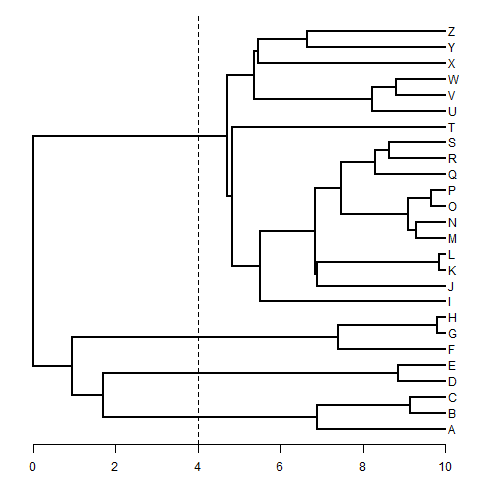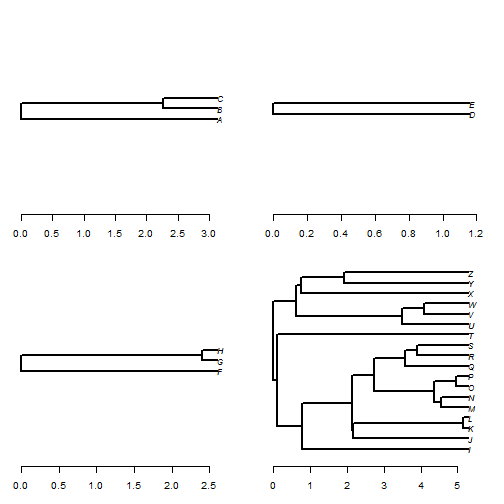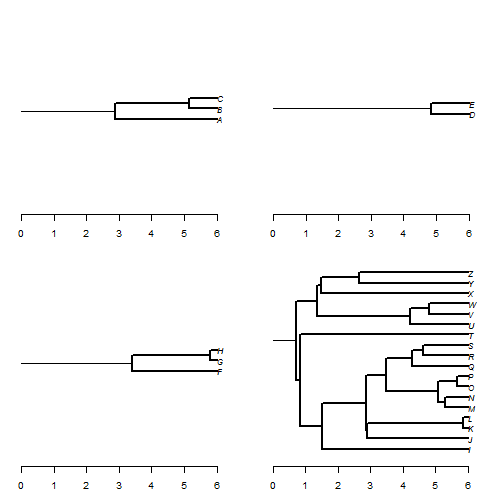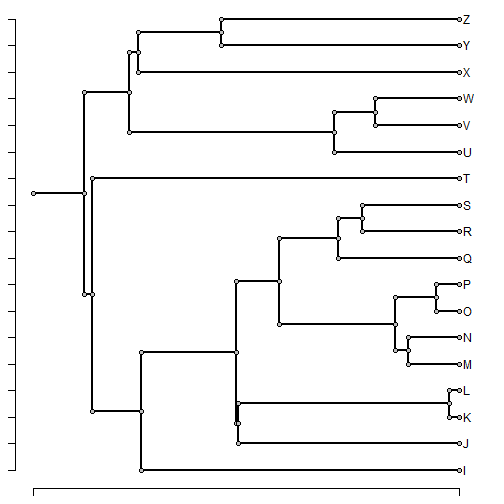## Monday, February 8, 2016

### `treeSlice` and the element `root.edge` for storing the stem length of a phylogeny (vs. other approaches)

The following question appeared recently on the phytools Comments Page:

I have been using your function 'treeSlice' to section a tree at different time intervals (5mya, 10mya, etc). However, the subtrees are collapsed onto the crown, so that a subtree cut at 5mya will have an age of 2my, because the first 3my is a single branch. Do you know of a way to either prevent this from happening (so the subtrees are not collapsed onto the crown) or, after the fact, append a branch at the root (to “restore” the stem)?

Actually - what `treeSlice` (which cuts the tree at a particular height & returns all subtrees) does with the stem is it includes it as the element `root.edge`. Let me show you what I mean:

``````library(phytools)
plotTree(tree,mar=c(4.1,1.1,1.1,1.1))
axis(1)
lines(c(4,4),par()\$usr[3:4],lty="dashed")
````````````obj<-treeSlice(tree,4,trivial=FALSE) ## all non-trivial subtrees
print(obj,details=TRUE)
``````
``````## 4 phylogenetic trees
## tree 1 : 3 tips
## tree 2 : 2 tips
## tree 3 : 3 tips
## tree 4 : 18 tips
``````

OK, now let's plot them:

``````N<-sapply(obj,Ntip)
par(mfrow=c(2,2))
for(i in 1:length(obj)){
par(mar=c(3.1,1.1,1.1,1.1))
plot(obj[[i]],edge.width=2,
y.lim=c((N[i]-max(N))/2,(max(N)-N[i])/2+N[i]))
axis(1)
}
``````(The part `y.lim=c((N[i]-max(N))/2,(max(N)-N[i])/2+N[i])` is just to ensure that all plotted trees have the same vertical scale.

At first glance, it would appear that we have indeed thrown away the stem; however if we change our script just a tiny bit to also plot the `root.edge` we can see that this is where that information has been stored:

``````par(mfrow=c(2,2))
for(i in 1:length(obj)){
par(mar=c(3.1,1.1,1.1,1.1))
plot(obj[[i]],edge.width=2,
y.lim=c((N[i]-max(N))/2,(max(N)-N[i])/2+N[i]),
root.edge=TRUE,lwd=2)
axis(1)
}
``````Now all of our trees have depth `6`, just as we expected.

For better or for worse, however, most functions that work with objects of class `"phylo"` ignore the root edge. For kicks I just added the optional argument `root.edge` to the functions `nodeHeights` and `nodeheight`. So, for example:

``````## shows height above the MRCA
nodeHeights(obj[])
``````
``````##          [,1]     [,2]
## [1,] 0.000000 3.118255
## [2,] 0.000000 2.264904
## [3,] 2.264904 3.118255
## [4,] 2.264904 3.118255
``````
``````## shows total height including root edge length
nodeHeights(obj[],root.edge=TRUE)
``````
``````##          [,1]    [,2]
## [1,] 2.881745 6.00000
## [2,] 2.881745 5.14665
## [3,] 5.146650 6.00000
## [4,] 5.146650 6.00000
``````
``````## height of all subtrees:
h<-sapply(obj,function(x) max(nodeHeights(x)))
h
``````
``````##  3.118255 1.153572 2.596285 5.279221
``````
``````## with root edge
h<-sapply(obj,function(x) max(nodeHeights(x,root.edge=TRUE)))
h
``````
``````##  6 6 6 6
``````

Finally, another conceivable way to store a root edge is as an extra singleton node in the tree. Consequently, I added the following function to convert a tree with a root edge to a tree with a singleton node:

``````rootedge.to.singleton<-function(tree){
root.edge<-if(!is.null(cw\$root.edge)) cw\$root.edge else 0
cw\$edge[which(cw\$edge>Ntip(cw))]<-cw\$edge[which(cw\$edge>Ntip(cw))]+1
cw\$edge<-rbind(Ntip(cw)+c(1,2),cw\$edge)
cw\$Nnode<-cw\$Nnode+1
cw\$edge.length<-c(root.edge,cw\$edge.length)
cw
}
``````

Try it on the biggest subtree from before:

``````singleton.tree<-rootedge.to.singleton(obj[])
plotTree.singletons(singleton.tree)
``````Unfortunately, relatively few functions in R can work with a `"phylo"` object containing singleton nodes.

These phytools updates can be seen here.

That's all for now.

#### 1 comment:

1.That's great, thanks very much.

Note: due to the very large amount of spam, all comments are now automatically submitted for moderation.